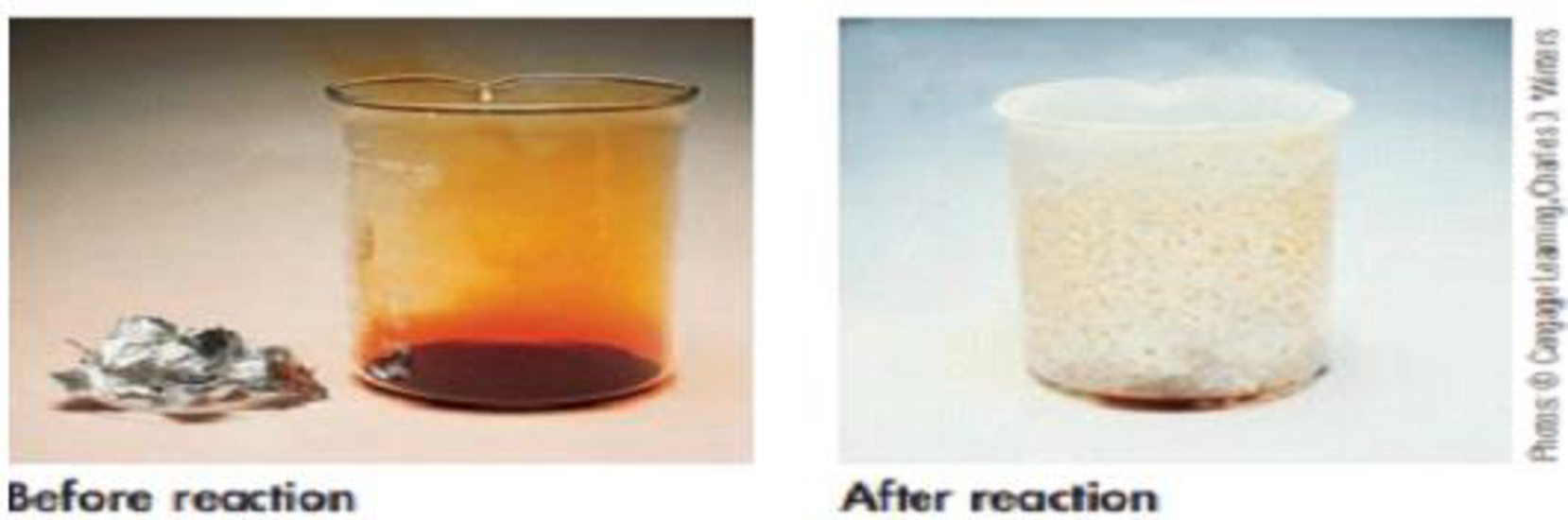Chapter 4, Problem 3PS

Chapter
Section
Textbook Problem

Like many metals, aluminum reacts with a halogen (here the orange-brown liquid Br2) to give a metal halide, aluminum bromide. (The white solid on the lip of the beaker at the end of the reaction is Al2Br6.)2 Al(s) + 3 Br2(ℓ) → Al2Br6(s)What mass of Br2, in grams, is required for complete reaction with 2.56 g of Al? What mass of white, solid AI2Br6 is expected?

Interpretation Introduction

Interpretation:

From the given reaction the mass of Bromine in gram is required to react completely with 2.56g of Al  and the mass of Al2Br6 (in gram) produced has to be determined.

Concept Introduction:

Numberofmole=GivenmassofthesubstanceMolarmass

• The molar mass of an element or compound is the mass in grams of 1 mole of that substance, and it is expressed in the unit of grams per mol (g/mol).
• For chemical reaction balanced chemical reaction equation written in accordance with the Law of conservation of mass.
• Law of conservation of mass states that for a reaction total mass of the reactant and product must be equal.
• Stoichiometric factor is a relationship between reactant and product which is obtained from the balanced chemical equation for a particular reaction.
Explanation

Balanced chemical equation for the given reaction is,

2Al(s)+3Br2(l)Al2Br6(s)

The amount (moles) of Al  available can be calculated by using the equation

Numberofmole=GivenmassofthesubstanceMolarmass

2.560gAl ×1molAl 26.982gAl =0.09487molAl

Using the stoichiometric factor calculating the amount of Bromine required based on the amount of Al  as follows,

0.09487molAl×3molBr21molAl=0

Still sussing out bartleby?

Check out a sample textbook solution.

See a sample solution

The Solution to Your Study Problems

Bartleby provides explanations to thousands of textbook problems written by our experts, many with advanced degrees!

Get Started